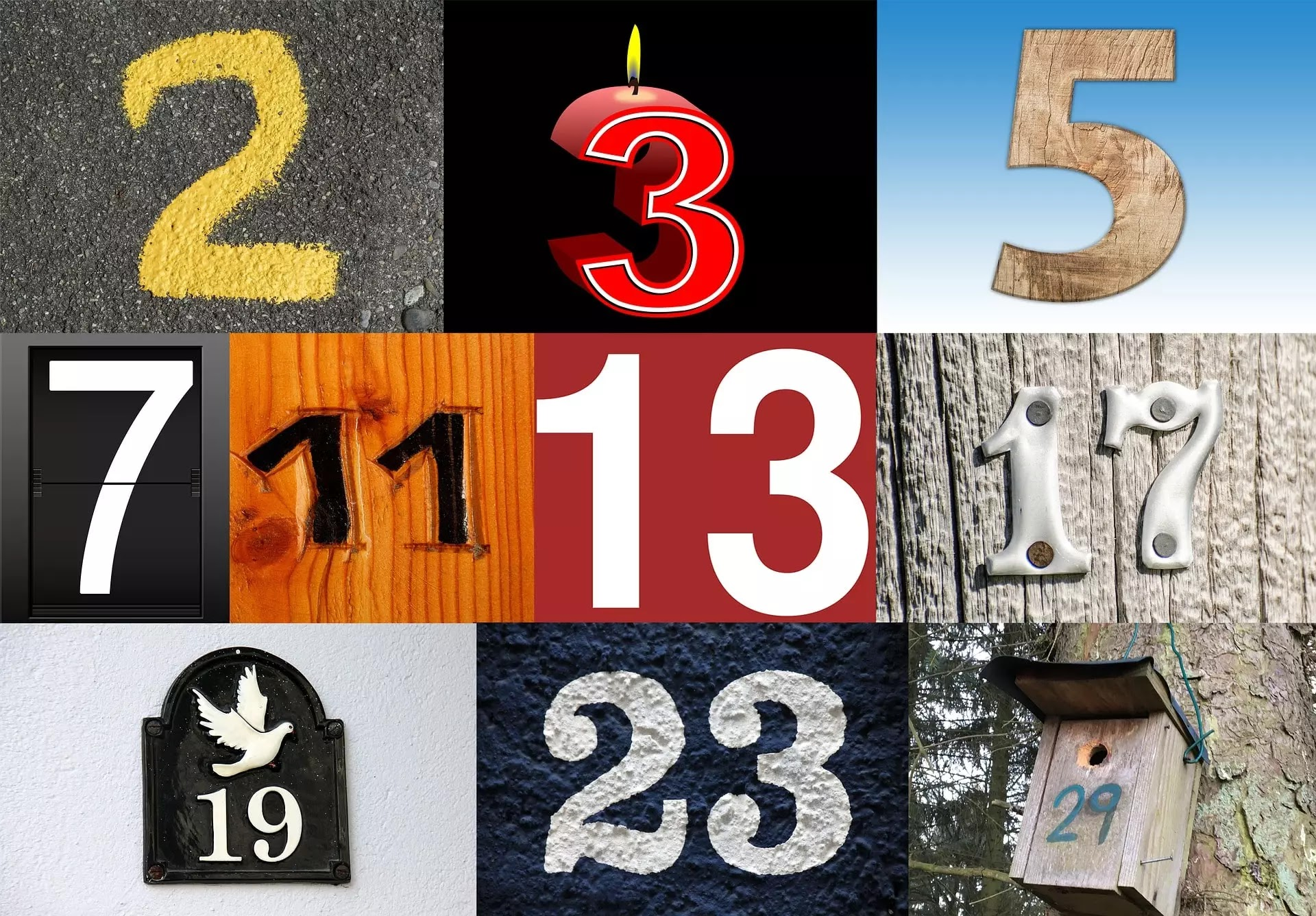Best Data Structures and Algorithm Course Get now!

# C++ program to print prime numbers [New]

In this program, we will make a c++ program to print prime numbers up to n. We will also print prime numbers between 1 and 100.

In this program, we will make a c++ program to print prime numbers upto n.

• Prime numbers up to n
• Prime numbers between 1 to 100
• Prime numbers in a given range

Basic knowledge required: for-loop & if-else.

## C++ program to print prime numbers upto n

We have to print prime numbers from 1 to n, where n is the upper range. The user gives the upper range according to their choice.

```#include<iostream> using namespace std; int main() {     int n,i,j;     cout<<"Enter upper range:";     cin>>n;     cout<<"\n Prime Numbers from 1 to "<<n<<": ";     for(i=1;i<=n;i++)     {         for(j=2;j<=i;j++)         {              if(i%j==0)             break;         }     if(i==j)     cout<<j<<" ";     } return 0; }
```

Output:
Enter upper range:50
Prime Numbers from 1 to 50: 2 3 5 7 11 13 17 19 23 29 31 37 41 43 47## Print prime numbers between 1 and 100

This program will print prime numbers from 1 to 100.

`#include<iostream> using namespace std; int main() {     int i,j;     cout<<"\n Prime Numbers from 1 to 100: ";     for(i=1;i<=100;i++)     {         for(j=2;j<=i;j++)         {             if(i%j==0)             break;         }     if(i==j)     cout<<j<<" ";     } return 0; }`
Output:
Prime Numbers from 1 to 100: 2 3 5 7 11 13 17 19 23 29 31 37 41 43 47 53 59 61 67 71 73 79 83 89 97

## C++ program to print prime numbers in a given range

In this program, we have to display prime numbers in a given range, i.e., lower and upper limits. The lower limit, as well as the upper limit, is provided by the user according to their choice.

`#include<iostream>using namespace std;int main(){    int l,u,i,j;    cout<<"Enter lower limit:";    cin>>l;    cout<<"Enter upper limit:";    cin>>u;    cout<<"\n Prime Numbers from "<<l<<" to "<<u<<": ";    for(i=l;i<=u;i++)    {        for(j=2;j<=i;j++)        {            if(i%j==0)            break;        }    if(i==j)    cout<<j<<" ";    }return 0;} `
Output:
Enter lower limit:10
Enter upper limit:50
Prime Numbers from 10 to 50: 11 13 17 19 23 29 31 37 41 43 47

## Find Prime Numbers From 1 to N

Given a number N, find number of primes in the range [1,N].
```#include<bits/stdc++.h>
using namespace std;
int checkPrime(int n)
{
//factors of 10 are 1,2,5,10
/* 10-1*10, 2*5,5*2,10*1
so we can run loop from 1 to sqrt(n)*/
//factors of 7 - 1,7
//for prime numbers, there are only two factors
int c=0;
for(int i=1;i*i<=n;i++)
{
if(n%i==0)
{
if(i*i==n)
c+=1;
else
c+=2;
}
}
if(c==2)
return true;
return false;
}
int main(){

int n;
cin>>n;
int count=0;
for(int i=1;i<=n;i++)
{
if(checkPrime(i))
{
count++;
}
}
cout<<count<<endl;
return 0;
}
```
Input: 6
Output: 3
There are three prime numbers from 1 to 6 {2,3,5}.